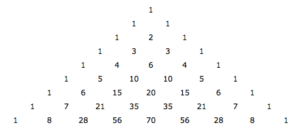# Pascal’s Patterns

What is the sum of each row? Find a way to describe the pattern of sums by comparing each row’s sum to the corresponding row number.Extension
What other patterns do you notice in this triangle of numbers?